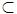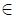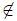Problems

# Partition of the set

Consider a set consisting of the first n natural numbers: Nn = {1, 2, ..., n}. Partition - a representation of this set as the union of one or more non-empty sets. Examples of partitions for n = 5 are:

{1, 2, 3, 4, 5} = {1, 2, 3} U {4, 5}

{1, 2, 3, 4, 5} = {1, 3, 5} U {2, 4}

{1, 2, 3, 4, 5} = {1, 2, 3, 4, 5}

{1, 2, 3, 4, 5} = {1} U {2} U {3} U {4} U {5}

In total there are 52 partitions of N5. Note that the partitions that differ only by order of the merged sets did not differ.

Partition of the set Nn can be ordered lexicographically.

In order to determine the order, first define the lexicographic order on subsets of Nn. We say that a setANnlexicographically less than the setBNn and write A < B, if one of the following statements:

• There existsi, such thatiA, iB, for all j < i: jA iff jB, and there is ak > i, such thatkB;
• AB and i < j for all iA and jB \ A.

It is obvious that this relation is a total order on subsets of Nn. Now we define the canonical representation of the partition as a representation in that combines multiple lexicographically.

Partitions are ordered lexicographically as follows. Partition ofNn = A1 U A2 U ... U Aklexicographically less than the partition ofNn = B1 U B2 U ... U Bl, if there is an i, asA1 = B1, A2 = B2, ..., Ai-1 = Bi-1 and Ai < Bi.

According to the partition of Nn find the following in the lexicographic order decomposition.

Input

The input file contains several descriptions of the tests. Each description is the canonical representation of the partition. The first line contains the description of n and k - number of elements in the partitions the set and the number of parts in a partition (1n200). Subsequent k rows contain elements of the partition. Elements of each set in ascending order.

Describe the tests are separated by blank lines. The last line of input contains two zero. This test should not be handled.

Sum of n over all the descriptions do not exceed 2000.

Output

For each test, the following output in lexicographic order decomposition. If the partition in the input file is the latest in leksigoraficheskom order, display the first in lexicographic order. Use the same format as the input file. Separate partitions from each other by blank lines.

Time limit 1 second
Memory limit 64 MiB
Input example #1
5 2
1 2 3
4 5

5 2
1 3 5
2 4

5 1
1 2 3 4 5

5 5
1
2
3
4
5

0 0

Output example #1
5 2
1 2 3 4
5

5 4
1 4
2
3
5

5 2
1 2 3 5
4

5 4
1
2
3
4 5